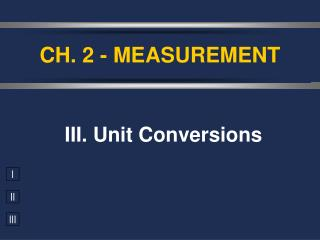DownloadDownload PresentationCH. 2 - MEASUREMENT

# CH. 2 - MEASUREMENT

Download Presentation## CH. 2 - MEASUREMENT

- - - - - - - - - - - - - - - - - - - - - - - - - - - E N D - - - - - - - - - - - - - - - - - - - - - - - - - - -
##### Presentation Transcript

1. CH. 2 - MEASUREMENT III. Unit Conversions

2. A. Dimensional Analysis • The “Factor-Label” Method • Units, or “labels” are canceled, or “factored” out

3. A. Dimensional Analysis • Steps: 1. Identify starting & ending units. 2. Line up conversion factors so units cancel. 3. Multiply all top numbers & divide by each bottom number. 4. Check units & answer.

4. A. Dimensional Analysis • Lining up conversion factors: = 1 1 in = 2.54 cm 2.54 cm 2.54 cm 1 = 1 in = 2.54 cm 1 in 1 in

5. B. Conversion Factors • Conversion Factors to Know: • 1 in = 2.54 cm • 1 mile = 2,580 ft • 1 lb = 454 g • 1 kg = 2.2 lbs • 1 L = 1.06 qt

6. mega- kilo- 1 M(u) = k(u) = 103 (u) 106 (u) BASE UNIT deci- (u) = 1(u) = 100 101 d(u) centi- 1 (u) = 102 c(u) milli- 1 (u) = 103 m(u) micro- 1 (u) = 106 (u) nano- 1 (u) = 109 n(u) pico- 1 (u) = 1012 p(u) B. Conversion Factors Prefix Conversion Factor

7. cm in  C. Practice Problems 1) Your European hairdresser wants to cut your hair 8.0 cm shorter. How many inches will he be cutting off? 8.0 cm 1 in 2.54 cm = 3.2 in

8. 2) A light bulb requires 0.032 amps to operate. What is this quantity in milliamps? A mA C. Practice Problems 0.032 A 103 mA 1 A = 32 mA

9. qt mL C. Practice Problems 3) How many milliliters are in 1.00 quart of milk? 1 L 1.057 qt 1000 mL 1 L 1.00 qt = 946 mL

10. cm pieces C. Practice Problems 4) A piece of wire is 1.3 m long. How many 1.5-cm pieces can be cut from this wire? 1 piece 1.5 cm 100 cm 1 m 1.3 m = 86 pieces

11. 5) A road race is 805 dm long. What is the length of the race in kilometers? dm km C. Practice Problems 1 m 102dm 1000 km 1 m 805 dm = 8.05 x 10-2 km

12. cm yd C. Practice Problems 6) The Eagles needs 550 cm for a 1st down. How many yards is this? 1 ft 12 in 1 yd 3 ft 1 in 2.54 cm 550 cm = 6.0 yd

13. in3 L C. Practice Problems 7) How many liters of water would fill a container that measures 75.0 in3? 1 L 1000 cm3 (2.54 cm)3 (1 in)3 75.0 in3 = 1.23 L

14. lb cm3 C. Practice Problems 8) You have 1.5 pounds of gold. Find its volume in cm3 if the density of gold is 19.3 g/cm3. 1 cm3 19.3 g 1 kg 2.2 lb 1000 g 1 kg 1.5 lb = 35 cm3